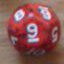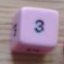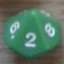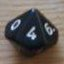An expression will not work and I can't figure out why

## An expression will not work and I can't figure out why

 Mon Sep 16 02:02:10 2019   by   Vine So I am new to the Troll language and have been struggling to make the following expression workx:=1d8;sum(accumulate x:=x@1d8 until (count x)=2)Now, this specific form works correctly. However, setting "until (count x)=" to anything other than 2 causes "An error during calculation". I would expect this behaviour with count x=1, but not with count x=3 or more, yet it does return an error with values of 3 or moreAs far as I understand, "x:=x@1d8" means that size of set x is increased by 1 with every pass. So when we enter the cycle, set x has 1 member in it. After the first pass set x has 2 members in it. After the second pass set x has 3 members in it and so on. So if count x=1, cycle's termination condition would never become true so I would expect an error. However, with count x=3 I expect the cycle to run once, termination condition is still false, but then the second pass occurs and termination condition is now true and we would have rolled a d8 3 times. Yet instead I am getting an error, so what have I gotten wrong here? Mon Sep 16 10:09:04 2019   by   Torben There are actually two different variables called x in the expression, it is in fact equivalent to`x:=1d8;sum(accumulate y:=x@1d8 until (count y)=2)`which, unsurprisingly, gives a count of 2 every time. The reason is that accumulate introduces a new variable which is visible only in the until/where clause.The accumulate and repeat expressions are somewhat limited: The defined variable is calculated from scratch in every iteration and can not use its previous value.  This allows some optimisations.  To emulate a general while loop, you must use function definitions and recursion.But if you add one d8 in every iteration and want to stop at a particular count n, why not just write it as `sum n d8` ? Mon Sep 16 13:19:59 2019   by   Vine Oh okay, so that's why it wasn't working. Thanks, I guess I'll try an approach with some functions As for why not just write sum 3d8, what I am actually trying to so us calculate a specific lvl 11 fighter damage per round against AC 1u, which requires three attacks, each of which is a conditional, so I figured a while loop would be a good solution, but before I can write a while loop with a bunch of conditionals, I need to figure out how to write a while loop that rolls a d8 3 times. Tue Sep 17 10:00:38 2019   by   Torben If it is just three conditional rolls, the easiest approach is probably to just write three if-then-else expressions -- no need for a loop.